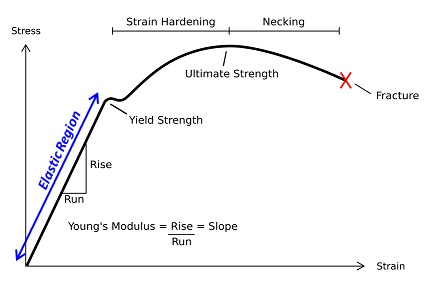# Difference Between Stress and Strain

## Main Difference – Stress vs. Strain

When deforming forces act on an object, they can change the object’s shape. The main difference between stress and strain is that stress measures the deforming force per unit area of the object, whereas strain measures the relative change in length caused by a deforming force.

## What is Stress

Whenever a force attempts to deform an object, we say that the object is under stress. Stress is defined as the deforming force per unit area of the object. Since we can resolve any force on an object into directions parallel and perpendicular to the surface, we define normal stress to be equal to the force perpendicular to the surface per unit area. Similarly, we define shear stress as the force parallel to the surface  per unit area. If the force acting on a surface is$F$ and the area of the surface is$A$, then the stress$\sigma$ is given by:$\sigma=\frac{F}{A}$

Stress has the same dimensions as pressure, so the units used for measuring stress are also N m-2 or Pa (1 Pa=1 N m-2). When forces act to lengthen the material, the stress is referred to as tensile stress. When the forces try to compress a material, the stress is referred to as compressive stress.

## What is Strain

Strain measures the amount of relative deformation caused by a force acting on an object. For simplicity here, we will only consider the normal strain, created by normal stress. Suppose the original length of the object is$l_0$ and due to stress, the length changes to$l_1$. The change in length is$\Delta l=l_1-l_0$. The strain$\epsilon$ is then given by,$\epsilon =\frac{\Delta l}{l_0}$

Since strain is given by a fraction where the numerator and denominator both have units of length, the strain itself has no units. i.e. it is a “dimensionless quantity”. It is common to see strain expressed in terms of percentages.

## Stress vs. Strain Curve

We can draw a graph of how the strain in a body changes as we vary the stress acting on the object (this can be done, for example, by adding weights). These graphs, called stress vs. strain curves, reveal lots of information about the nature of the material that the object is made of. The figure below shows the typical stress-strain curve for a ductile material (“ductile” means that the material can be stretched out well):Stress-strain curve for a ductile material

The gradient of the elastic region of the curve is called the Young Modulus. This is a very important number for materials engineers, as it gives how much strain would be caused by a given stress in a material.

## Difference Between Stress and Strain

### What it Measures

Stress gives the force acting per unit area of an object.

Strain gives the relative change in length due to deforming forces.

### Units

Stress is measured in pascals (Pa).

Strain has no units; it is simply a ratio.

Image Courtesy

“Typical Stress vs. Strain diagram for a ductile material (e.g. Steel).” by Breakdown (Own work) [CC BY-SA 3.0], via Wikimedia Commons (Modified)